# Laser Induced Breakdown Spectroscopy Analysis¶

## Tutorial 2: LIBS Analysis using a Spreadsheet¶

### Introduction¶

\begin{aligned} I = X Y \frac{g_k A_{ki} C}{U(T)} K(a,x) e^{\frac{-E_k}{kT}} \end{aligned}

In this Tutorial, we will utilise the LIBS Equation from the previous Tutorial, to begin to analyse some samples.

For this work, we will use some spectra obtain from OREAS Geological Samples. To facilitate the analysis the samples were:-

• pressed into pellets - to reduce the variability in plasma temperature by ensure that the level of dust was kept at a minimum (pressed) and the sample surface was kept uniform (pellets)

• subjected to multiple laser pulses across the sample surface - to obtain an average spectra for analysis

### Importing the data¶

For this work we will use the following samples, and analyse for Sodium (Na)

Sample Na. Conc (ppm)
OREAS45e 594
OREAS501b 20848
OREAS601 14543
OREAS921 6068
OREAS603 4283
OREAS933 1515
OREAS903 301

The spectra were exported from the LIBS software as CSV files and opened in Excel.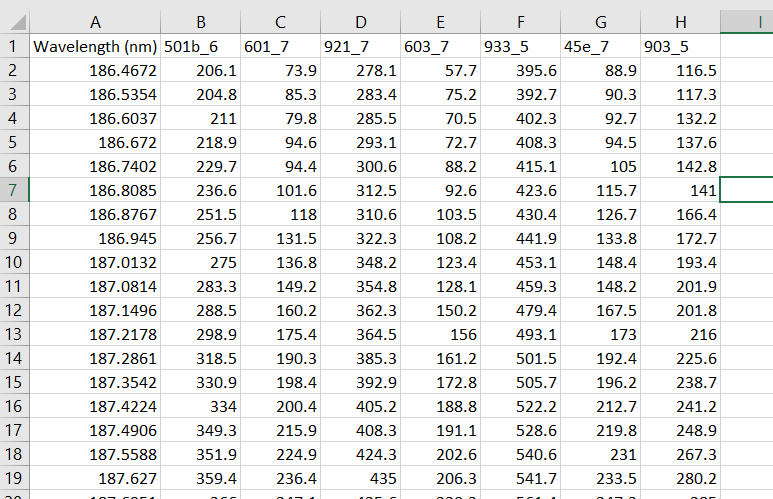Next we do a simple scatter line plot to get an overall view of our spectra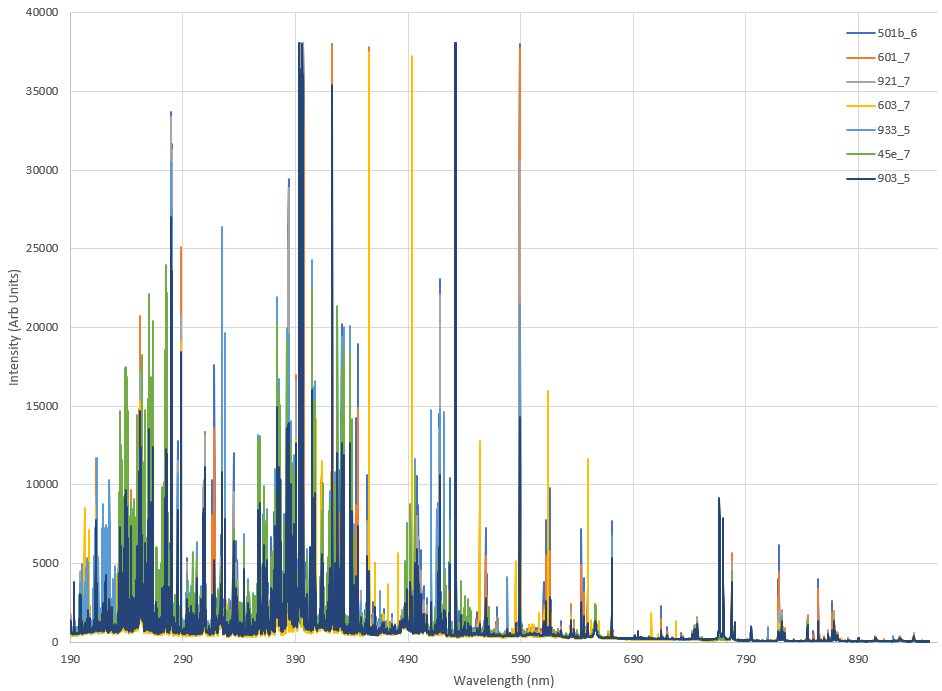This is a moderately complex LIBS spectra. Some early things to notice

• Some of the peaks between 390-590nm end abruptly at about 38000 counts. These are likely to have been too intense for the detection system. They won't have caused any damage, but they won't be any use for analysis purposes.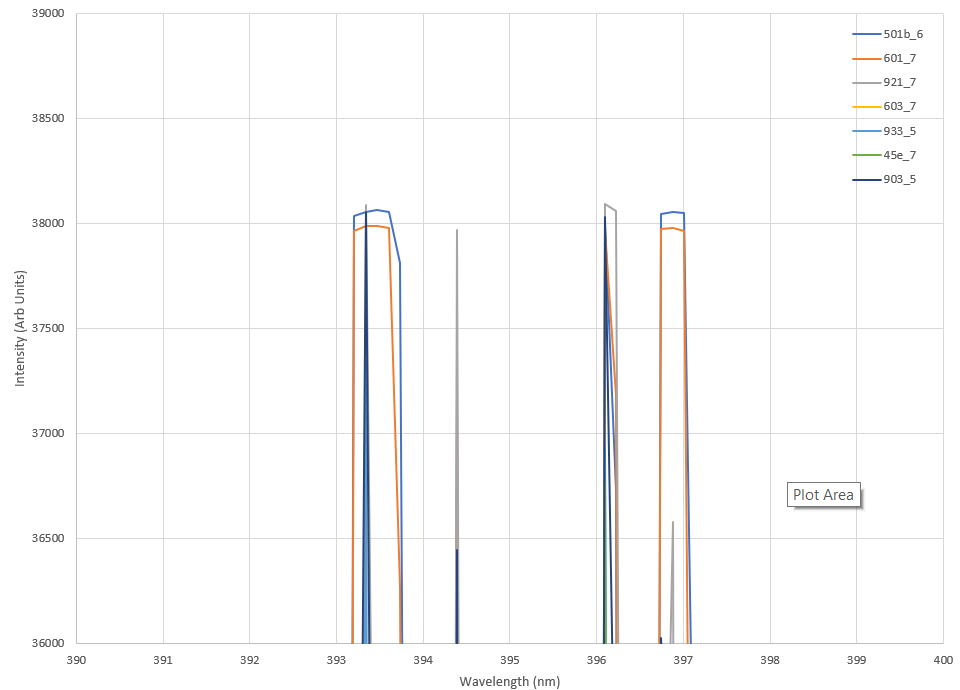• There are numerous peaks that are not identical across the samples - so there is a reasonable chance we will be able to measure the differences between the spectra, which makes quantitative measurements possible

• As discussed in the first Tutorial, this spectra is the combination of the unique spectra of all the elements in the sample. With time, a LIBS user knows the peaks that are unique for elements of interest and is able to examine a spectra such as the above and state that there is Hydrogen, Potassium, Sodium, Calcium, Aluminium, ... present.

## Analysing for Sodium¶

### Finding the Sodium Peaks¶

For most people doing this tutorial, they are not yet at the stage where they can reel off the wavelengths for the 3 or 4 strongest/favourite peaks for an element.

Instead we will use a reference website. As discussed last Tutorial, NIST and AtomTrace both provide LIBS Spectra resources, but we will use the resources at libs-info.com as they provide a list in intensity order.The peaks at 588.995nm, 589.592nm, 819.48nm and 818.33nm look worth investigating. There may also be signal at 568.82nm and 330.24nm, but these are probably ~1000 times weaker, and in parts of the spectrum with peaks from other elements.

### 589nm and 589.59nm Peaks (Intensity)¶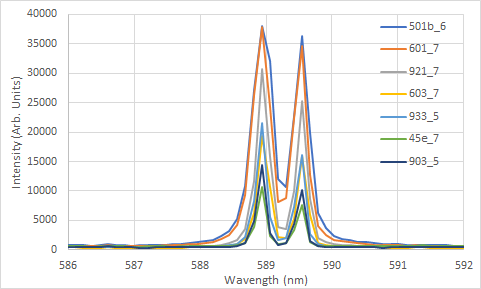Narrowing down the focus to just the peaks at 589/589.5nm shows that peaks are well separated/resolved from other peaks, but the 589nm peak (and possibly the 589.5nm) are close to that 35000+ Intensity that indicates detector saturation.

Wavelength (nm) 501b 601 921 603 933 45e 903_5
586.9689 731.8 648.9 494.8 357.6 476.9 563.9 405.8
587.0917 747.7 667 491.6 363.7 492.5 559.6 395
587.2146 791.4 684.6 494.2 362.1 493.8 584.8 390
587.3375 812.3 703.7 513.5 372.3 472.2 582.6 413
587.4603 868.6 743.3 519.7 365.2 482.5 578.7 403.4
587.5831 930.6 784.2 544.9 382.6 481 568.5 411.8
587.7059 1001.6 830.2 559.7 387.3 509.7 591.3 410.3
587.8287 1117.9 925.5 568 408.2 493.1 594.1 420
587.9515 1253.1 1006.8 630.9 440.1 539.2 596.4 445.9
588.0742 1427 1163.7 643.5 447.8 535.2 610.5 425.5
588.197 1753.9 1393.7 725.5 483.1 582.8 606.4 450.4
588.3197 2285.8 1795.5 882.3 552.9 682.5 644.1 490.3
588.4424 3246.7 2554.9 1123.8 661.7 751.6 651.4 522.3
588.5651 5196.8 4150.5 1702.1 927.2 1019.6 743.3 646.7
588.6877 10779.7 9293.7 3552.4 1833.5 2197.9 1196.9 1236.1
588.8104 27090 26295.4 11703.8 6185.8 8317.5 3883.4 4873.4
588.933 38021.8 37788.1 30650.2 19405.8 21514.3 10650.6 14327.8
589.0557 32012.9 24295.9 15719.5 10647.9 5616 2399.1 2920.8
589.1783 12066.3 8170.9 3826.1 2203.2 1593.9 918.5 849.4
589.3009 10610.8 8723.2 3577.7 1954.8 2072.7 1128 1194.1
589.4235 22700.8 22375.6 10041.7 5299 7315.3 3441 4307
589.546 36270.7 34549.4 25271.4 15950.2 16094.8 7597.5 10203.2
589.6686 19859.4 12638.9 8563 5865.4 2774.8 1314.4 1453.8
589.7911 6243.6 4054.2 2051.6 1213 920.4 730.7 617.2
589.9136 3633.4 2474.4 1336.4 822.7 735.4 697 568.7
590.0361 2431.9 1737.8 971.1 612.7 637.4 628 467
590.1586 1910.4 1427 846.7 538.6 597.3 646.7 448.6
590.281 1659.5 1257 742.2 512.2 600.4 634.1 450.5
590.4034 1413.7 1097 711.7 477.9 571.6 643.2 441.2
590.5259 1266.9 986.1 679 468.3 584.1 647.9 438.5
590.6483 1134.1 893.5 609.3 468.2 541.7 607.4 422.2
590.7707 1011.4 853.4 591 472.3 528.3 616.5 400.8
590.8931 974.3 810 561.2 400.4 533.4 624.5 423.1
591.0154 916.8 778.9 551.8 376.2 547.2 628.1 430.9
591.1378 865.2 735.5 549.5 396.2 557.2 646.5 417.1
591.2601 880.2 746.9 567.9 408.8 617.5 737.1 456.3
591.3824 872.9 725.9 609.4 417.9 648 742.2 471.2
591.5047 790.4 654.1 542 378.2 533.9 642.4 422.9
591.627 759.4 657.9 519.8 370.3 517.5 633.9 423.3
591.7492 747.3 674.2 509.9 363.1 489.5 634.9 433.2
591.8715 741.6 632.8 506.1 376 507.7 626.8 418.9
591.9937 728.9 640.2 521.6 367.9 508.2 619.7 424.7
592.1159 747.7 647.2 509.8 365.3 496.1 623.2 448.8

Choosing the highlighted rows in the table (as these are closest to the peak wavelengths and correspond to the top of the peaks gives us the following)

Sample Na Conc (ppm) 589nm (raw) 589.5nm (raw) 587.5nm (raw) 589nm (net) 589.5nm (net)}
OREAS45e 594 10650.6 7597.5 568.5 10082.1 7029
OREAS501b 20848 38021.8 36270.7 930.6 37091.2 35340.1
OREAS601 14543 37788.1 34549.4 784.2 37003.9 33765.2
OREAS921 6068 30650.2 25271.4 544.9 30105.3 24726.5
OREAS603 4283 19405.8 15950.2 382.6 19023.2 15567.6
OREAS933 1515 21514.3 16094.8 481 21033.3 15613.8
OREAS903 301 14327.8 10203.2 411.8 13916 9791.4

While it doesn't show in the plot, there is a slightly different baseline for each of different samples. To correct for that, we choose a wavelength without an peaks (here we choose 587.5nm) and subtract this value from the peak heights.

The LIBS Equation, assuming we keep the Temperature constant for all the plasmas can be reduced to

\begin{aligned} I = X Y \frac{g_k A_{ki} C}{U(T)} K(a,x) e^{\frac{-E_k}{kT}} = b C \end{aligned}

and traditionally this relationship would be preserved by plotting concentration on the X axis and Intensity on the Y Axis. However, for analysis we are most interested in Concentration, so we will plot concentration on the Y axis and Intensity on the X Axis and look for a fit for

\begin{aligned} C = \frac{1}{b} I = f I \end{aligned}Neither curve is what we might have hoped for. The sharp increase in slope shows that the intensity is not increasing very much for the increase in concentration, suggesting that the detector is saturating.

### 589nm and 589.59nm Peaks (Peak Area)¶

Alternatively to Intensity, we can take peak area as our measure. Starting with the LIBS Equation.

\begin{aligned} \int_{\lambda_{lower}}^{\lambda_{upper}} I d \lambda = \int_{\lambda_{lower}}^{\lambda_{upper}} X Y \frac{g_k A_{ki} C}{U(T)} K(a,x) e^{\frac{-E_k}{kT}} d \lambda = b C \int_{\lambda_{lower}}^{\lambda_{upper}} d \lambda = {constant_0 \quad constant_1 \quad } C \end{aligned}

or, in words, we can create linear calibration curves plotting the peak area vs concentration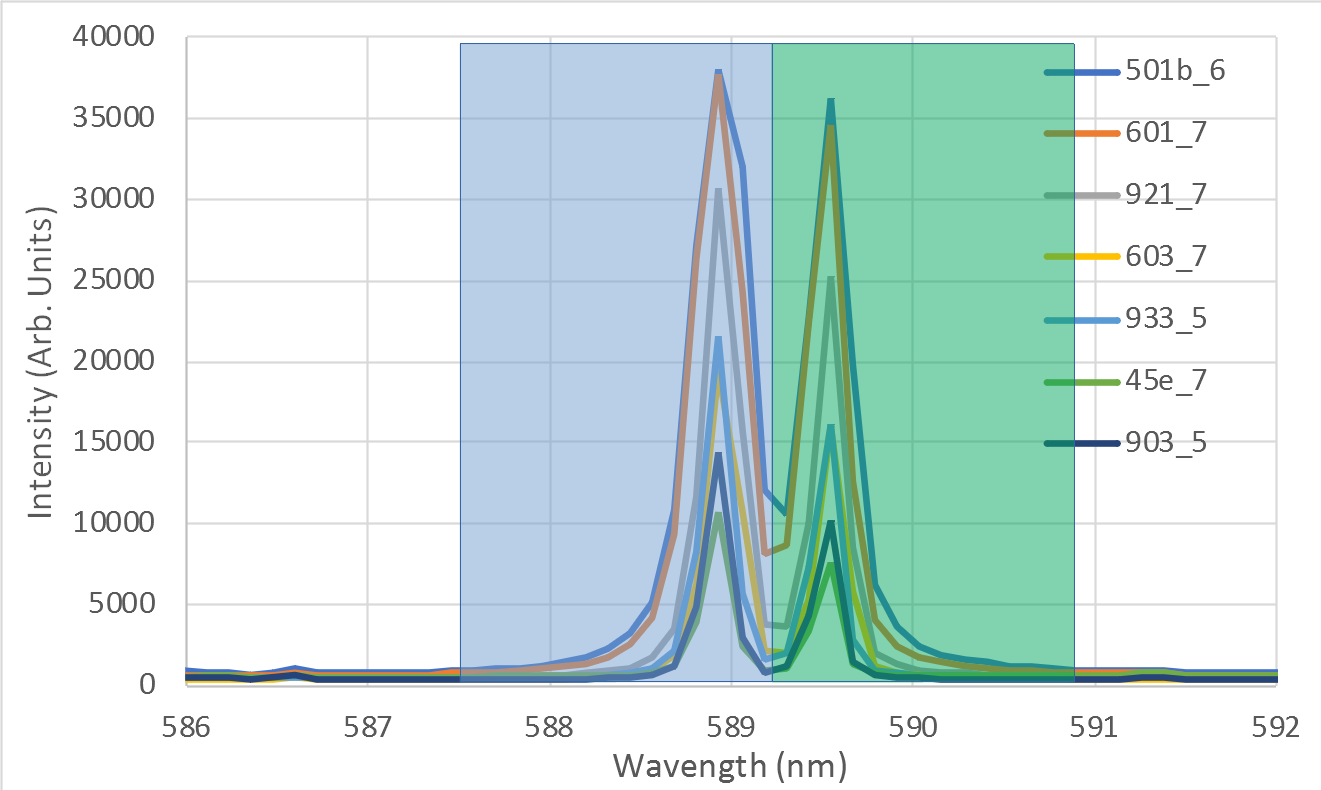So if we add up the area under the curve (which is equivalent to adding up the individual intensities for this small sample), then that will give the intensity sum under the total curve. As with the peak intensities, we should subtract off the 'pedestal' (the peak area offset) to get a net peak area.

In this case, because there are no detectable peaks for any other elements between the 589nm and 589.5nm peaks, we can also investigate the area of the two peaks combined.

Sample Na Conc (ppm) 589nm Peak Area 589.5nm Peak Area Both Peaks together
OREAS45e 594 17823.5 11790 29815.7
OREAS501b 20848 135766.5 101219.2 223851.3
OREAS601 14543 118193.4 85745 192873.4
OREAS921 6068 68781.8 49618.6 114770.5
OREAS603 4283 41565.5 30225.4 69756.9
OREAS933 1515 40173 27801.2 66853.7
OREAS903 301 23859.6 16422.1 39287.4

Again, plotting concentration on the y axis, peak area this time on the x axis givesThe $R^{2}$ values show a significant improvement, although probably still not as good as we might have hoped.

To note, while the peak intensities showed saturation, this is significantly less present in the peak areas making them often a better candidate for calibration curves.

### 818.3nm and 819.4nm Na Peaks¶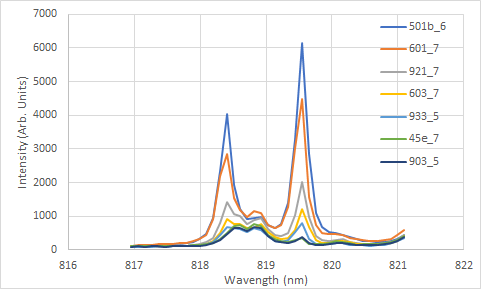For comparison, let's look at the Na peaks in the 817-821nm region. The Na Peaks at 818.3nm and 819.4nm are quite well resolved, but there is a peak from an unidentified element in the 818.8nm region.

Repeating the analysis using the Peak Intensity gives

Sample Na Conc (ppm) 818.3nm (raw) 819.4nm (raw) 817.5nm 818.3nm (net) 819.4nm (net)
OREAS45e 594 502.6 345.8 107.1 395.5 238.7
OREAS501b 20848 4049.6 6160.1 142.9 3906.7 6017.2
OREAS601 14543 2855.1 4487.6 164.1 2691 4323.5
OREAS921 6068 1417.5 2024 117.2 1300.3 1906.8
OREAS603 4283 920.4 1217.5 110.5 809.9 1107
OREAS933 1515 675.3 801 95.3 580 705.7
OREAS903 301 477.3 377.1 90.6 386.7 286.5

Which gives the following calibration curves for Peak Intensity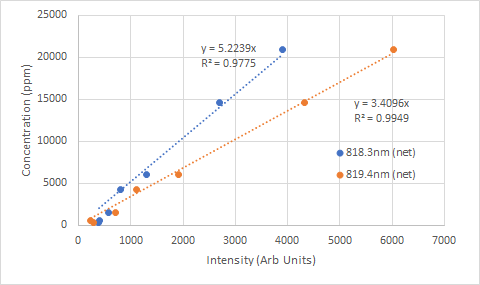These are considerably more linear than the equivalents around 588nm, with $R^{2}$ or 0.97 or better.

For Comparison, the peak areas are

Sample Na Conc (ppm) 818.3nm 819.4nm
OREAS45e 594 2628.6 991.5
OREAS501b 20848 11246.5 15645.2
OREAS601 14543 9285.6 11479.6
OREAS921 6068 4936 4942.7
OREAS603 4283 3364.6 2979.2
OREAS933 1515 2812.6 1923
OREAS903 301 2477.1 1061.5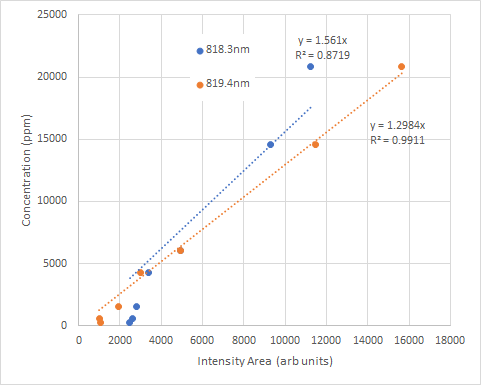In this case, the Peak Area calibration curve $R^{2}$ are still quite respectable, but not as good as the Peak Intensity curves.

## Analysis Performance¶

While a $R^{2} > 0.95$ is nice to have, in practice what is more important is the analytical performance of our calibration curve.

In these tutorials we will look at a range of methods, two comparatively simple measures are

• detection limit - estimates the smallest concentration that can be detected
• linear regression uncertainty - estimates uncertainty in measurement along the calibration curve

### Detection Limit (or Limit of Detection = LOD)¶

Convention uses the figure of $3 \sigma$, where $\sigma$ is an estimate of the noise in the spectra, as the intensity of the smallest feature that can be detected.

While hardly a proof, the image below shows peaks that are increasing multiples of $\sigma$. The background using noise with a gaussian distribution with a standard deviation of 0.5. The peak at $3 \sigma$ is only just the strongest peak shownReturn to our equation above, where we have included the units for f

\begin{aligned} C = \frac{1}{b} I = f (units = \frac{Concentration}{Intensity}) I \end{aligned}

we note that our smallest peak that we are confident is above the noise represents a concentration of

\begin{aligned} LOD = f (units = \frac{Concentration}{Intensity}) 3 \sigma = 3 \sigma (slope) \end{aligned}

This makes intuitive sense, the calibration curve with the greatest change in intensity for the smallest change in concentration (that is, with the least slope of a plot of concentration (y axis) vs intensity (x axis) will have the smallest LOD.

Note: the traditional formula of $\frac{3 \sigma}{slope}$ applies when the calibration curve plots intensity (y axis) vs concentration (x axis)

There are a number of ways to calculate $\sigma$. It is important that the noise is measured near the analysis peak (as the noise can change across the spectrum, particularly if different spectrometers/optica fibres/systems are used to measure different wavelenght regions).
In the calculations here, the standard deviation of the intensity values in the spectrum of the lowest concentration sample (OREAS903) over a range without any obvious peaks - 817-817.8nm for the peaks at 818/819nm and 587-588nm for the peaks at 589nm.

### Standard Error (of calibration curve)¶

Alternatively, the Standard Error of the Regression (S) provides a measure of the typical difference between the predicted concentration (from the calibration curve) and the actual - as measured by the points in the curve. Mathematically, it is defined as

$S = \sqrt{ \frac{\sum \left( C - f I \right)^2 } {N-1}}$

### Evaluating the Performance (Peak Intensity)¶

For the Peak Intensity calibration curves the performance is

Peak Sigma Slope $R^2$ LOD (ppm) Standard Error (ppm)
818.3nm 7.83 5.22 0.9775 122.6 1181.4
819.4nm 7.83 3.41 0.9949 80.0 562.0
589nm 15.93 0.34 0.5889 16.3 5054.2
589.5nm 15.93 0.41 0.719 19.4 4178.2
589nm (ignore highest conc.) 15.93 0.25 0.721 11.96 3411.1
589.5nm (ignore highest conc.) 15.93 0.31 0.5992 14.8 2846.2

Some things to note

• The Standard Error is not also the calibration curve with the best Limit of Detection
• As noted previously, the calibration curves at 589nm and 589.5nm so some flattening of the curve (likely due to detector saturation), removing the peak of highest concentration improves the performance of the curves (lower LOD and better Standard Error)

### Evaluating the Performance (Peak Area)¶

For the Peak Area calibration curves the performance is

Peak Sigma Slope $R^2$ LOD (ppm) Standard Error (ppm)
818.3nm 7.83 1.56 0.8719 36.6 2821.3
819.4nm 7.83 1.30 0.9911 30.5 743.4
589nm 15.93 0.126 0.8809 6.0 2720.5
589.5nm 15.93 0.173 0.8985 8.3 2511.3
589nm+589.5nm 15.93 0.0766 0.878 3.7 2753.4
589nm (ignore highest conc.) 15.93 0.104 0.8644 5.0 1983.7
589.5nm (ignore highest conc.) 15.93 0.145 0.8785 6.9 1877.9
589nm+589.5nm (ignore highest conc.) 15.93 0.063 0.8572 3.0 2036.2

Noting:

• $\sigma$ is the same, but the peak areas are much larger, leading to better LODs
• However, the average difference between the calibration curve is worse, which is reflected in the Standard Error (and the $R^2$)

## Best Calibration Curve?¶

So, after all this work - what is the best calibration curve to use?

In this case, the best depends on the question to be answered.

If the question is, what calibration curve will give the lowest detection limits? Then the answer is using the calibration curve based on the peak area of the combined 589nm and 589.5nm peaks.

If the question is, what calibration curve will give the best overall perfomance? (that is, the expected difference between the calibration and the 'true' value) Then using the calibration curve based on the peak intensity of the 819.4nm peak is the best answer.

What is the best calibration curve will be a question we will keep asking throughout this series.